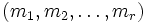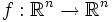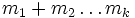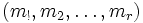# Parabolic function

Let$(m_1,m_2,\ldots,m_r)$ be a tuple of integers whose sum is$n$. Then, a function$f:\R^n \to \R^n$ is said to be of parabolic type$(m_1,m_2,\ldots,m_r)$ if for any$k \le r$, the effect on the first$m_1 + m_2 \ldots m_k$ coordinates is independent of the values of the remaining coordinates.
If$f$ is also continuously differentiable, this is equivalent to the condition that the Jacobian matrix of$f$ be of parabolic type$(m_!,m_2,\ldots,m_r)$.